# Elastic Potential Energy

The amount of elastic potential energy (EPE) stored in a string is given by the following equation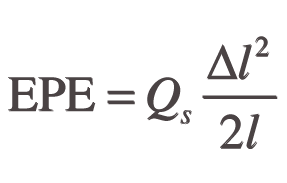Where Δl is the extension resulting from the tensioning of the string and the plucking action, l is the natural length of the string and Qs is the net elasticity or ‘relative strength’ of the wire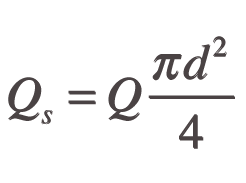Where

Q = Young's modulus
d = diameter

To calculate the amount of energy stored in a harp string with its known parameters when under tension the natural length l of the string must be established first in order to calculate the elongation Δl.

Let

Δl1 = the elongation resulting from the initial string tension
Δl
2 = the elongation resulting from the plucking action
Δl = ??Δl1+Δl2 = total elongation
l = the natural length of the string
l1 = the vibrating length of the string under tension

From Hooke’s Law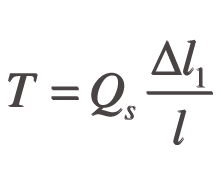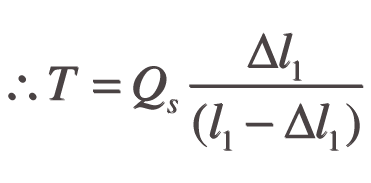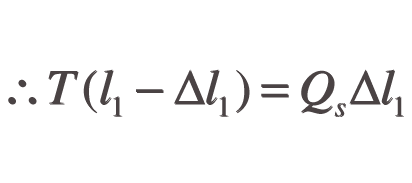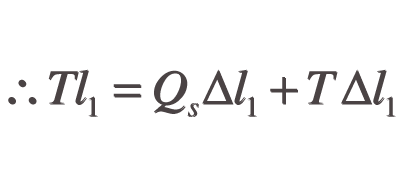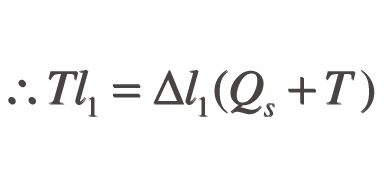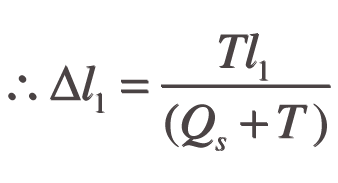Values for Qs (Q and d), l1 and T are known for any given string, allowing Δl1 to be calculated and therefore l, which is equal to l1-Δl1

The elongation resulting from the plucking action Δl2 can be calculated thus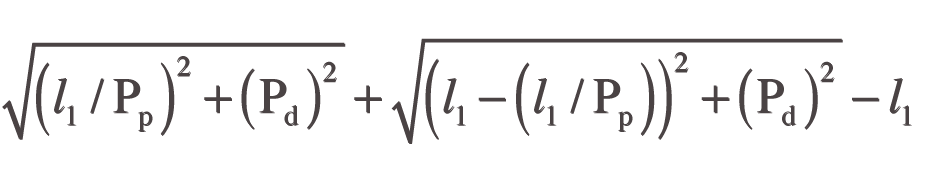Where Pp is the plucking point and Pd is the plucking depth.